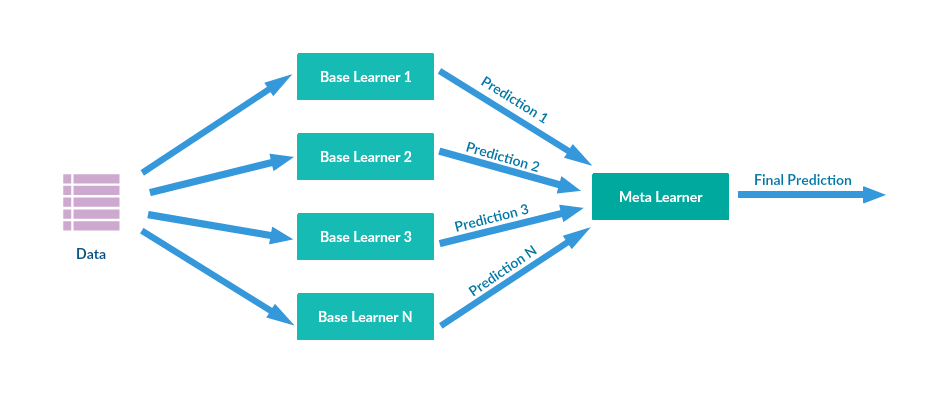Join thousands of AI enthusiasts and experts at the Learn AI Community.

## PublicationLatest

# Machine Learning Model Stacking in Python

Last Updated on January 21, 2022 by Editorial Team

#### Author(s): Suyash Maheshwari

Originally published on Towards AI the World’s Leading AI and Technology News and Media Company. If you are building an AI-related product or service, we invite you to consider becoming an AI sponsor. At Towards AI, we help scale AI and technology startups. Let us help you unleash your technology to the masses.

#### Find out how stacking can be used to improve model performance

Stacking is a type of ensemble learning wherein multiple layers of models are used for final predictions. More specifically, we predict train set (in CV-like fashion) and test set using some 1st level models, and then use these predictions as features for 2nd level model.

We can do it in python using a library called ‘Vecstack’. The library has been developed by Igor Ivanov and was released in the year 2016. In this article, we will have a look at the basic implementation of this library for a classification problem.

The library can be installed using :

`pip install vecstack`

Next, we import it :

`from vecstack import stacking`

First, we will create individual models and perform hyperparameter tuning to find out the best parameters for all of the models. In order to avoid overfitting, we apply cross-validation split the data into 5 folds, and compute the mean of roc_auc score.

1. Decision Tree Classifier :
`#Hyperparameter tuning for decision tree classifier`
`clf = DecisionTreeClassifier()parameters={'min_samples_split' : np.arange(10,100,10),'max_depth': np.arange(1,20,2)}clf_random = RandomizedSearchCV(clf,parameters,n_iter=15 , scoring = 'roc_auc' , cv =5 , verbose = True)clf_random.fit(x_train, y_train)`
`#Best parameters {'min_samples_split': 70, 'max_depth': 9}`
`#mean roc_auc score0.8142247920534071`

Similarly,

2. Random Forest Classifier :

`#Best parameters{'min_samples_split': 90, 'max_depth': 9}`
`#mean roc_auc score0.8051500705643935`

3. Multilayer Perceptron Classifier :

`#Best parameters{'max_iter': 100, 'learning_rate': 'constant', 'hidden_layer_sizes': (20, 7, 3), 'activation': 'tanh'}`
`#mean roc_auc score0.8017714839042659`

4. KNeighbours Classifier :

`#Best parameters{'weights': 'distance', 'n_neighbors': 7}`
`#mean roc_auc score0.7013120709379057`

5. Support Vector Machine Classifier :

`#Best parameters{'max_iter': 700}`
`#mean roc_auc score0.8672302452275072`

Next, we create a base layer for our stacking model bypassing all of the above-mentioned models. We want to predict train set and test set with some 1st level models, and then use these predictions as features for 2nd level models. Any model can be used as a 1st level model or a 2nd level model.

`# 1st level models`
`models = [KNeighborsClassifier(n_neighbors= 3) ,            DecisionTreeClassifier(min_samples_split= 70,max_depth=9),           RandomForestClassifier(min_samples_split= 90,max_depth=9),          MLPClassifier(max_iter= 100, learning_rate='constant',hidden_layer_sizes= (20, 7, 3), activation= 'tanh') ,           LinearSVC(max_iter= 700) ]      S_Train, S_Test = stacking(models,                                              x_train, y_train ,x_test ,                              regression=False,                              mode='oof_pred_bag',                                   needs_proba=False,                                    save_dir=None,                                        metric= roc_auc_score,                                n_folds=4,                                             stratified=True,                                       shuffle=True,                                         random_state=0,                                        verbose=2)`

Next, we pass the predictions of these models as input to our layer 2 models which is the MLP classifier in this case. We also perform Hyperparameter Tuning and Cross-Validation for this model.

`mlp = MLPClassifier()parameters = {'hidden_layer_sizes':[(10,5,3), (20,7,3)], 'activation':['tanh', 'relu'], 'learning_rate':['constant', 'adaptive'], 'max_iter' :[100, 150]}mlp_random = RandomizedSearchCV(mlp,parameters,n_iter=15 , scoring = 'roc_auc' , cv =5 , verbose = True)mlp_random.fit(S_Train , y_train)grid_parm=mlp_random.best_params_print(grid_parm)print(mlp_random.best_score_)`

The mean of the Roc_Auc score for the final model is 0.9321746030659209.

Conclusion :

Therefore, using stacking we were able to improve the performance of the model by at least 7%! Stacking is a way to combine the strengths of multiple models into a single powerful model. Having said this, Stacking may not always be the best thing to do as it involves significant use of computational resources and the decision to use it must be decided based on the business case, time and money. A huge shoutout to Igor Ivanov, developer of this library, for such amazing work.Machine Learning Model Stacking in Python was originally published in Towards AI on Medium, where people are continuing the conversation by highlighting and responding to this story.

Join thousands of data leaders on the AI newsletter. It’s free, we don’t spam, and we never share your email address. Keep up to date with the latest work in AI. From research to projects and ideas. If you are building an AI startup, an AI-related product, or a service, we invite you to consider becoming a sponsor.

Published via Towards AI